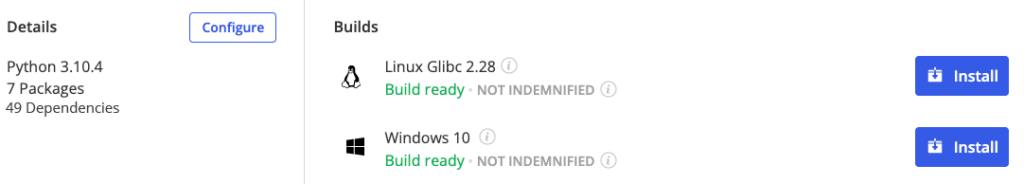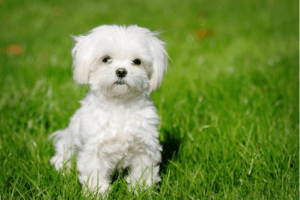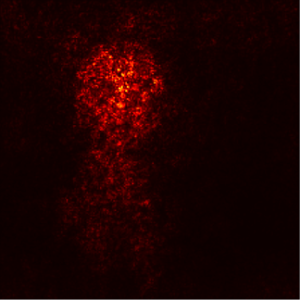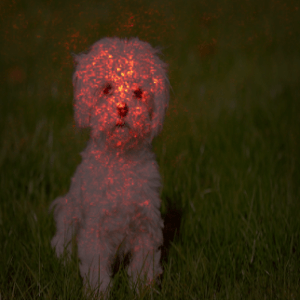# How Neural Networks Work

728 VIEWS

One of the problems with Machine Learning (ML) and Deep Learning (DL) is the so-called black box aspect, which is the fact that it’s often difficult to ascertain how the routine arrives at its decisions. One way to help users understand how image classification routines arrive at their conclusions is to show which parts of the image are weighted more than others. For example, when working with a convolutional neural network (CNN) image, you can show which parts of the image are important to the CNN when performing the classification. One way to do this is to use saliency maps.

Saliency maps aid in quickly determining which parts of an image influenced the neural network’s classification output. It answers the question, “What does the model look for at the pixel level when classifying a given image?”

There are numerous examples of ML routines taking the simplest route to image classification, including:

• Determining an animal is a horse based on copyright watermarks on the pictures of horses that were not present on other animal photos.
• Because different hospitals specialize in different injuries, a CNN was found to be predicting diagnoses based on a hospital’s ID, which is determined by annotations on x-rays.

In other words, in some cases, deep learning isn’t really all that deep. But more and more they’re being relied on for everything from driving our cars to diagnosing our diseases. How can we be sure that ML is reliable and unbiased? Continue reading to learn one way to visualize how neural networks think by employing saliency maps.

## Visualizing How Neural Networks Think Using Saliency Maps

In this post, we will use the backpropagation algorithm, which is one of the most popular and straightforward methods for obtaining a saliency map with a pretrained CNN classification. Using backpropagation, we can highlight pixels in the input image based on the amount of gradient they receive, thus revealing their impact on the final classification score.

In a world where we have easy access to cutting-edge neural network models, it is pointless to build our own. There are several pretrained CNN models that we can use to simulate how neural networks think. To improve our accuracy rates, we will use the existing, pretrained VGG-19 ConvNet implemented in PyTorch and assisted by the torchvision module.

VGG-19 is a 19-layer CNN that was trained on more than a million images from the ImageNet dataset. The network was trained on images with rich feature representations. As a result, it is known to be capable of categorizing images into thousands of different object categories. Andrew Zisserman and Karen Simonyan of the University of Oxford built and trained the VGG-19 network in 2014, and it was published in 2015. For colored images, the pretrained network requires an image size of 224 x 224 pixels as input.

We will follow these steps to visualize how neural networks think and interpret them using saliency maps:

1. Set up the deep learning model
2. Open the image
3. Preprocess the image
5. Visualize the results

All set? Let’s dive into the code.

## Before You Start: Install The Neural Network Visualization Python Environment

• A version of Python 3.10
• All the dependencies used in this post in a prebuilt environment for Windows and Linux

Or you can also use our State tool CLI to install the runtime environment and project code:For Windows users, run the following at a CMD prompt to automatically download and install the Neural Network Visualization runtime into a virtual environment:

`powershell -Command "& \$([scriptblock]::Create((New-Object Net.WebClient).DownloadString('https://platform.activestate.com/dl/cli/2080745363.1499703358_pdli01/install.ps1'))) -c'state activate --default Pizza-Team/Neural-Network-Visualization'"`

For Linux users, run the following to automatically download and install the Neural Network Visualization runtime into a virtual environment:

`sh <(curl -q https://platform.activestate.com/dl/cli/2080745363.1499703358_pdli01/install.sh) -c'state activate --default Pizza-Team/Neural-Network-Visualization'`

## Step 1. Import Libraries To Set Up The Deep Learning Model

If you’ve installed the Neural Network Visualization environment, the first step is to configure the deep learning model by importing the libraries that will be responsible for interpreting the pretrained VGG-19 model:

```#Setting up- Library and Namespaces Imports

import torch

import torchvision

import torchvision.transforms as T

import numpy as np

import matplotlib.pyplot as plt

from torchsummary import summary

import requests

from PIL import Image```

As noted above, we are using backpropagation to find gradients with respect to the VGG-19 network parameters. Therefore, we’ll set param.requires _grad to False:

```#Using pretrained VGG-19 model

model = torchvision.models.vgg19(pretrained=True)

for param in model.parameters():

## Step 2. Open The Image

First, we must download an input image to extract the saliency map. For our purposes, we’ll use this image of a dog. It will serve as a sample image which can be replaced by any image with the correct URL.

##source: https://specials-images.forbesimg.com

```def downloadImage(url,Imagename):

response = requests.get(url)

with open(Imagename,"wb") as f:

f.write(response.content)

# Using Python to open the image

img = Image.open('input.jpg')```

### Step 3. Preprocess the Image

1. Transforming it to the required size (224 x 224)
2. Converting it to a PyTorch tensor
3. Normalizing the tensor

The ToTensor function converts a PIL image or NumPy array (height x width x channels) in the range [0, 255] to a torch float tensor in the range [0, 1]. We can normalize the tensor using the ImageNet dataset’s mean and standard deviation.

All of this is implemented in the following code snippet:

```# Image preprocessing

def preprocess(image, size=224):

transform = T.Compose([

T.Resize((size,size)),

T.ToTensor(),

T.Normalize(mean=[0.485, 0.456, 0.406], std=[0.229, 0.224, 0.225]),

T.Lambda(lambda x: x[None]),

])

return transform(image)

#depreocess the image by backward transformation

def deprocess(image):

transform = T.Compose([

T.Lambda(lambda x: x),

T.Normalize(mean=[0, 0, 0], std=[4.3668, 4.4643, 4.4444]),

T.Normalize(mean=[-0.485, -0.456, -0.406], std=[1, 1, 1]),

T.ToPILImage(),

])

return transform(image)

def show_img(PIL_IMG):

'''Visualize the image output by plotting'''

plt.imshow(np.asarray(PIL_IMG))

#calling the preprocess function

X = preprocess(img)```

## Step 4. Retrieve The Gradient Using Saliency Maps

To find the gradient, we compute the variance in the maximum score value from the input image. Ideally, every input image should be in the RGB format, which references the pixel coloring. To derive a saliency class for each pixel (i,j), we need maximum magnitude across all color channels, as shown in the code below:

```# initial model evaluation

model.eval()

# to find the gradient change based on the input image

#computing the model scores and getting maximum index

scores = model(X)

score_max_index = scores.argmax()

score_max = scores[0,score_max_index]

#backward propagation of output

score_max.backward()

#Finding saliency

## Step 5. Visualize The Results

To visualize the results of our neural network, we can use a heatmap to plot the saliency map:

```# Plotting the saliency map as a heatmap

plt.imshow(saliency, cmap=plt.cm.hot)

plt.axis('off')

plt.show()```

Given the input image, you can literally point to the places where the ConvNet draws its output object, as shown in the heatmap image below:When you compare the original image to the heatmap above, you can see which parts of the image pixels are important for classification.Although we may not be able to obtain a direct classification variable to reveal the neural network black box, we can deduce how the results have been achieved. Therefore, saliency maps are an essential facet of deep learning for understanding and visualizing how neural networks think.

## Conclusions

Most machine learning models (like decision trees) make it very simple to deduce which variables contributed to the predictive results. However, as we progress to deep learning, it becomes more difficult to interpret the results due to the model’s complexity.

As ML gets incorporated into more and more aspects of our lives (whether we want it or not), more research along the lines of that demonstrated here will be vital to understanding how our world looks to an AI. Failure to do so could prove fatal. Greater understanding can hopefully lead to better models that won’t be so easy to fool with the addition of just a few pixels, or something entirely unexpected.

Saliency maps that have the ability to interpret CNN image results from the gradient of the output using the PyTorch framework are just one tool in the toolbox. Many more tools will need to be developed before we can have any real confidence that the AI we’re coming to rely on actually warrants our trust.Faith Kilonzi is a full-stack software engineer, technical writer, and a DevOps enthusiast, with a passion for problem-solving through implementation of high-quality software products. She holds a bachelor’s degree in Computer Science from Ashesi University. She has experience working in academia, fin-tech, healthcare, research, technology, and consultancy industries in both Kenya, Ghana, and in the USA. Driven by intellectual curiosity, she combines her passion for teaching, technology, and research to create technical digital content.